# C - scanf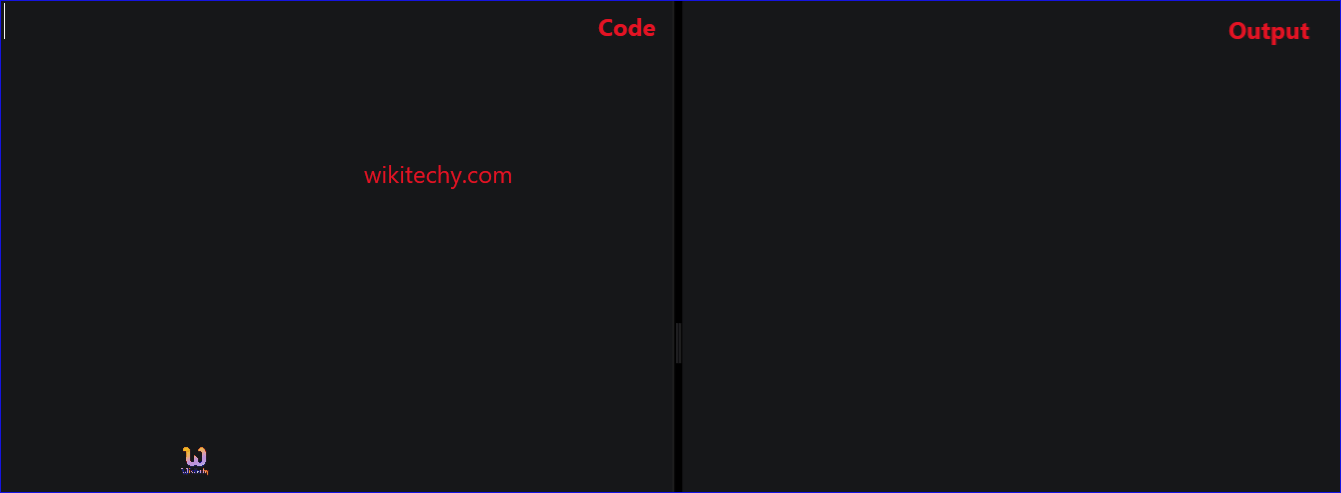Learn C - C tutorial - scanf - C examples - C programs

## C - scanf() Function - Definition and Usage

• In C -Language, scanf() is a predefined function in "stdio.h" header file. It can be used to read the input value from the keyword.
• The format string must be a text enclosed in double quotes.
• It contains the information for interpreting the entire data for connecting it into internal representation in memory.
• Example : integer (%d) , float (%f) , character (%c) or string (%s).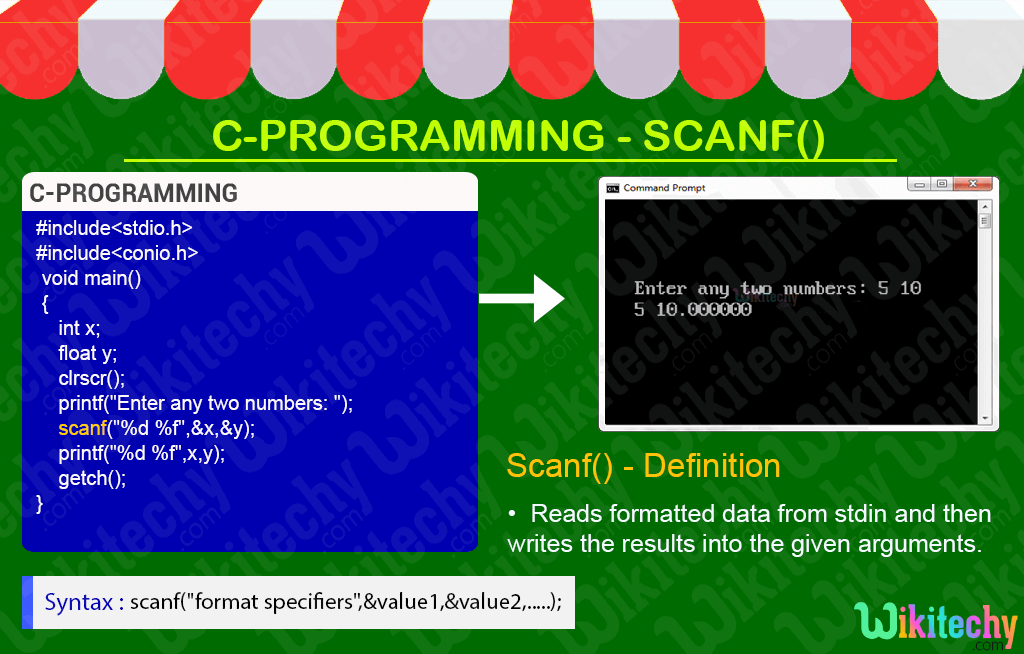## Format specifier :

Format specifier Type of value
%d Integer
%f Float
%lf Double
%c Single character
%s String
%u Unsigned int
%ld Long int
%lf Long double

## C Code - Explanation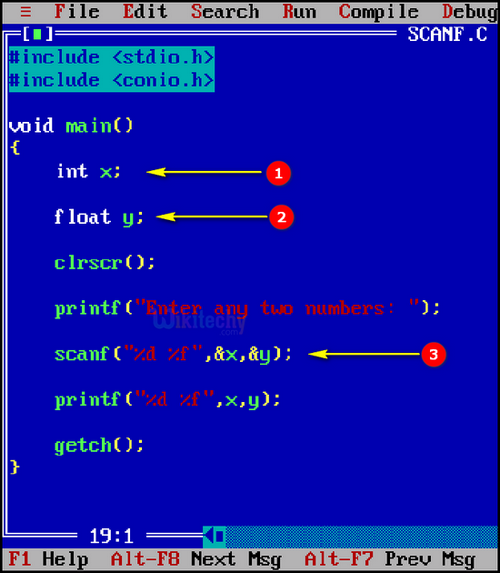1. In this example, “int x” specifies the integer value for the variable x.
2. Here, “float y” specifies the float value of the variable y.
3. Here scanf("%d %f",&x,&y) is being used to read the integer & float data from keyboard.
4. Here %d is an integer value of the variable x.
5. And %f is a float value of the variable y.

## Sample Output - Programming Examples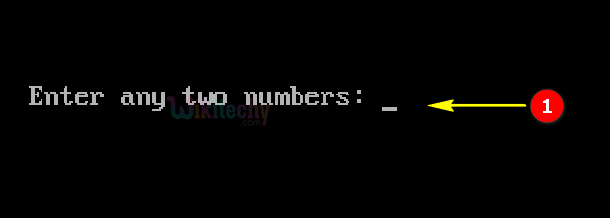1. Here in this output “Enter any two numbers: “ is represented in printf statement.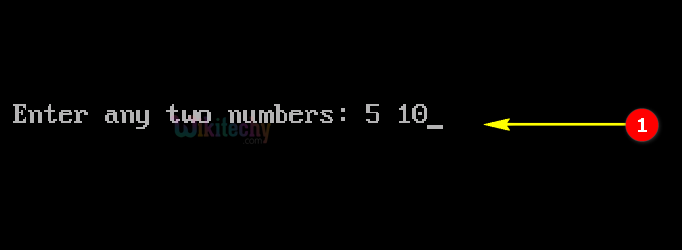1. Here in this output we are entering the values 5 and 10.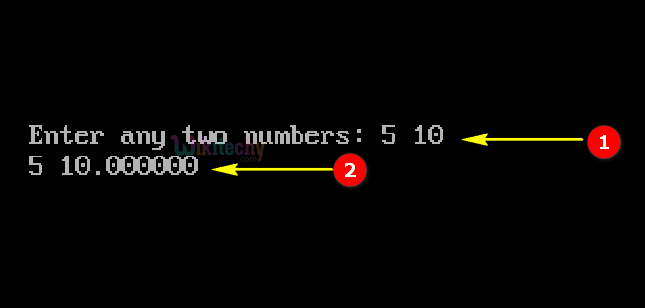1. Here in this output the entered values 5 and 10 are processed in the console window.
2. Here 5 is represented as integer value and 10.000000 as float value.